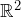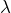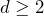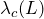# E. Broman: Higher-dimensional stick percolation

Abstract: The so-called stick percolation model was introduced by Roy in 1991. In its most basic form, the sticks are line-segments of lengths 1, the centers of the sticks are placed inaccording to a Poisson point process with intensity, while the orientations of the sticks are chosen uniformly and independently.

Since the introduction of this model, only a short mathematical note (by Popov and Vachkovskaia 2002) has been written on (an extension of) the model. However, within the physics and chemistry community there has been much more interest. In contrast to Roy’s model, the sticks there have a non-zero width and “live” in both two-dimensional and three-dimensional space.

In this talk, I consider two variants of the stick percolation model in any dimension. In both variants, the sticks have width 1 and length. In the first variant, the orientation distribution is uniform, while in the second variant, all sticks are oriented along the same direction.

Lettingdenote the critical intensity for the percolation phase transition of this model, the focus of this project has been to establish the asymptotic behavior ofasdiverges. For both variants, the exact scaling exponent has been obtained.

In the first part of the talk I will provide background material and state the main results. In the second part I will sketch the proofs.

## Video